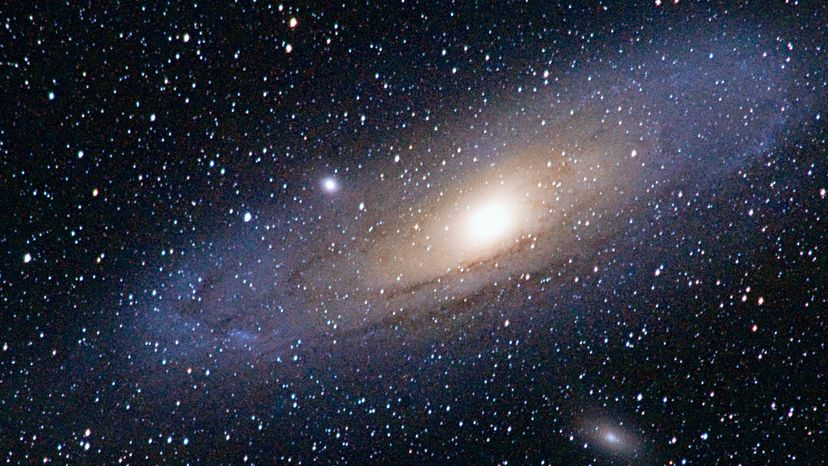# If you were to move all of the matter in the universe into one corner, how much space would it take up?No one truly knows how large the universe is. Tony Rowell / Getty Images

It's hard to answer this question exactly because there are some unknowns. But if you are willing to accept three assumptions, we can come up with an answer that sounds reasonable...

The first question is, "How big is the universe?" No one knows, but this Question of the Day assumes that the universe is a cube that is 30 billion light years on each side. That means that the whole universe contains about 2.7E+31 cubic light years.

The next question is, "How much matter does the universe contain?" The mass of the universe is a source of debate right now because there is no easy way to put the universe on a scale. This NASA page and this " Extension, Age and Mass of the Universe" article discuss different techniques that scientists use to estimate the mass. The latter article also includes an estimate of about 1.6E+60 kilograms for the mass of the universe. Other estimates give other numbers, but all are in that ballpark.

The next question is, "What density do you want to assume the mass will have once you push all of it into one corner?" Now, if you were really to do this -- if you actually did move all of the mass of the universe into one corner -- it would condense instantly into a black hole and potentially ignite another big bang. But let's say that you could keep it from doing that, and you were somehow able to keep all of the mass evenly distributed at the density of the sun. According to "Magnitudes of Physics", the density of the sun is about 1,410 kilograms per cubic meter. (For comparison, the density of water is 1,000 kilograms per cubic meter.)

If you are willing to accept these three assumptions, then:

### 1.1E+57 cubic meters of matter in the universe

A cubic light year contains about 1E+48 cubic meters. So all of the matter in the universe would fit into about 1 billion cubic light years, or a cube that's approximately 1,000 light years on each side. That means that only about 0.0000000000000000000042 percent of the universe contains any matter. The universe is a pretty empty place!